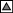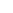MACROMEDIA FLASHACTIONSCRIPT: PROGRAMMATIC MOVEMENTin flash 3, the only way to move an object was with key frames and tweening. with the introduction of actionscript in flash 4, we can now retrieve and set an object's location, allowing us to move an object programmatically along a path. these examples show increasingly complex ways to move something around in flash 4 with actionscript. the first example shows how to move something along a path. the second shows how to redirect an object off a wall. the third example demonstrates simulated gravity and adds a speed limit to prevent the object from being drawn off screen. the last example is an experiment built on the components of the first three examples.

for information on programmatic motion in flash 5, see my time-based animation lecture notes from flash forward 2001 in san francisco.

as with the collision detection routines, thanks go to derek clayton and mike linkovich for their help with the math in these demos.

 > move an object along a path >>download the .fla (zipped) >>view the demo all of the movement systems presented here start out by initializing an object and its environment. for simple movement, initialization begins with placing the object randomly on the screen (this is done in the "init_object" subroutine). once the object is placed, we pick a random direction and speed for our object. in some languages, you might express the direction in radians (points on a circle) and speed as the distance travelled between each screen refresh (or time point). while this is an excellent method of tracking movement, it requires the use of sin, cos, and square root, which are not available in actionscript (but can be hand-calculated). since we have none of these math functions in actionscript (*sigh*), we express the speed and direction as a vector. a vector is made up of two points, a start point which is always defined by 0,0, and an end point which is defined by an arbitrary x and an arbitrary y coordinate. mathematically, a vector describes both direction (the angle of the line) and magnitude, (the distance between the two points). magnitude maps logically to speed in our case, because it represents the distance our object moves between screen refreshes. since we know the x and y position of our object, we can move it in any direction at any speed by simply incrementing the x and y by a standard amount (the defined vector), over and over. to get a starting vector, we pick a random x between -10 and 10, and a random y between -10 and 10 (in our code these values are stored as x_dir and y_dir, and are determined in the "getrandomDirection" subroutine). ```Set Variable: "max_dist" = "20" Set Variable: "x_dir" = Random ((max_dist) + 1) - (max_dist / 2) Set Variable: "y_dir" = Random ((max_dist) + 1) - (max_dist / 2) ``` then, every turn, we call the "move" subroutine, which updates the current location of the object by adding the vector (x_dir and y_dir) to it. ```Set Variable: "obj1_x" = GetProperty ("/obj1", _x) Set Variable: "obj1_y" = GetProperty ("/obj1", _y) Set Property ("/obj1", X Position) = obj1_x + x_dir Set Property ("/obj1", Y Position) = obj1_y + y_dir ``` > redirecting an object off a wall >>download the .fla (zipped) >>view the demo in this example, our ball has three new features: it bounces off the walls, it can be sized, and its speed can be controlled. before we can bounce our object off a wall, we first have to move it (using the "move" subroutine). we add the wall collision code to the "move" subroutine. once the ball is moved, we check its location to see if it has gone beyond its allowed boundaries (set as "leftmax", "rightmax", "topmax", and "bottommax"). let's take the left wall as an example to show how: if the x value of the ball's centre point plus its radius is less than the value of the left edge of the canvas (in the demo, zero), then the ball has exceeded the left wall. ```If (obj1_x < (leftmax + obj1_radius)) ``` when we know the ball has been moved outside of the boundaries, we have to recalculate the real location of the ball. in other words, if the ball had not gone through the wall, but had actually bounced off it, where would it be now? to find that out, we measure how far the ball travelled along the axis on which it exceeded the wall (this is stored in "amount_over"). then, we add or subtract that value to/from the wall's location and place the ball there. i suppose a diagram is probably in order:finally, we have to make the ball continue moving in the opposite direction at which it hit the wall. to do that we multiply the x_dir or y_dir (depending on whether the ball hit a wall on the x or y axis) by -1. put together, here's what it all looks like in code: ```If (obj1_x < (leftmax + obj1_radius)) Set Variable: "amount_over" = leftmax - obj1_x + obj1_radius * 2 Set Property ("/obj1", X Position) = amount_over Set Variable: "x_dir" = x_dir * -1 Else If (obj1_x > (rightmax - obj1_radius)) Set Variable: "amount_over" = obj1_x - rightmax + obj1_radius * 2 Set Property ("/obj1", X Position) = rightmax - amount_over Set Variable: "x_dir" = x_dir * -1 End If ``` to speed the ball up and slow it down, we increase the x_dir and y_dir by 150% or reduce them by 25%. these percentages are arbitrary. in the next example they're kept in a variable so you can easily set how much each speed change should affect the speed of the ball. there's a drawback to this method though: if you speed the ball up too much, you'll notice that it eventually can't be drawn on screen anymore. this is because when the ball is travelling a distance greater than the canvas with each move, repositioning it will always place it off the canvas again. in the next example you'll see how to prevent the ball from going that fast. to change the size of the ball, we increase or decrease the scale property in the "shrink" and "grow" subroutines. when shrinking the ball, we just need to be sure the reduction won't make the ball smaller than 0. ```If ((current_scale - scale_modifier) > 0) Set Variable: "current_scale" = current_scale - scale_modifier End If Set Property ("/obj1", X Scale) = current_scale Set Property ("/obj1", Y Scale) = current_scale ``` when expanding it, we need to be sure we aren't making the ball larger than the canvas size. because _scale is a percentage in actionscript, we have to do our check relative to the original height and width of the ball. ```If (obj1_origwidth * (current_scale/100) < (rightmax - 20) AND obj1_origheight * (current_scale/100) < (bottommax - 20) ) Set Variable: "current_scale" = current_scale + scale_modifier End If Set Property ("/obj1", X Scale) = current_scale Set Property ("/obj1", Y Scale) = current_scale ``` at then end of both operations, we recalculate the ball's radius so the collision with the walls will still be accurate. ```Set Variable: "obj1_radius" = GetProperty ("/obj1", _width) / 2 ``` > bouncing with gravity and a speed limit >>download the .fla (zipped) >>view the demo this demo adds two new features: gravity and a speed limit. to add gravity to the ball, we first make it bounce in a parabola by increasing the y_dir by a standard amount each time "move" is executed. if the ball is heading down (y_dir is positive), then it will move down faster as we incrementally increase y_dir until the ball bounces off the bottom, at which point, y_dir becomes a negative (it's moving up). when y_dir is negative, the ball will moves up slower, until y_dir is 0, then it will move down again faster. at this point there is no gravity because all the speed gained by y_dir on the way down is converted to speed up when the ball hits the bottom. similarly, though the ball loses its speed on the way up, it gains the exact amount it lost on the way back down. so, to make the simulated gravity pull the ball down more and more with each bounce, we also add friction to the bottom wall by reducing both the x_dir and y_dir by a standard percentage each time the ball collides with the bottom. instead of hard-coding the standard amount for the simulated gravity and friction, we use a variable, "grav_amount", to calculate how quickly the ball should lose speed. by setting grav_amount to 0, you can make the ball bounce forever. the max for grav_amount is 9.9. as grav_amount increases, it adds more to y_dir, and increases percentage of speed that is removed from the ball by the friction on the bottom wall. to add a speed limit, we put a check on the "speedUp" subroutine which prevents the ball from going further in one move on the x or y axis than the width or height of the bounded region minus the width of the ball. as the ball gets bigger, it is allowed to travel shorter and shorter distances. ```Comment: get the absolute values of x & y without sqrt. If (y_dir < 0) Set Variable: "y_dir_abs" = y_dir * -1 Else Set Variable: "y_dir_abs" = y_dir End If If (x_dir < 0) Set Variable: "x_dir_abs" = x_dir * -1 Else Set Variable: "x_dir_abs" = x_dir End If Comment: begin speedlimit check If (x_dir_abs * speed_modifier < rightmax - obj1_radius * 2) Set Variable: "x_dir" = x_dir * speed_modifier End If If (y_dir_abs * speed_modifier < bottommax - obj1_radius * 2) Set Variable: "y_dir" = y_dir * speed_modifier End If Comment: end speedlimit check ``` when we increase the size of the ball, we must also check to see that we're not making a large ball go at a speed that's only allowed for a smaller ball. if we find that that's happening, we have to reduce the speed of the ball to an allowable amount. ```If (obj1_origwidth * (current_scale/100) < (rightmax - 20) AND obj1_origheight * (current_scale/100) < (bottommax - 20) ) Set Variable: "current_scale" = current_scale + scale_modifier End If Set Property ("/obj1", X Scale) = current_scale Set Property ("/obj1", Y Scale) = current_scale Set Variable: "obj1_radius" = GetProperty ("/obj1", _width) / 2 Comment: check if we're going to fast for the ball's new size. If (y_dir < 0) Set Variable: "y_dir_abs" = y_dir * -1 Set Variable: "y_orientation" = "-1" Else Set Variable: "y_dir_abs" = y_dir Set Variable: "y_orientation" = "1" End If If (x_dir < 0) Set Variable: "x_dir_abs" = x_dir * -1 Set Variable: "x_orientation" = "-1" Else Set Variable: "x_dir_abs" = x_dir Set Variable: "x_orientation" = "1" End If Comment: begin speedlimit check If (x_dir_abs > rightmax - obj1_radius * 2) Set Variable: "x_dir" = ((rightmax - obj1_radius * 2) * .5) * x_orientation End If If (y_dir_abs > bottommax - obj1_radius * 2) Set Variable: "y_dir" = ((bottommax - obj1_radius * 2) * .5) * y_orientation End If Comment: end speedlimit check ``` > randomation: experimental bouncing demo >>view the demo this experiment adds sound, gravity controls, a squishing effect on bounces, and a random size/speed toggle. no .fla for this one...i have to keep *some* secrets to myself ;)
revision history
august 5, 1999: posted.
sept 17, 1999: added a link to a tutorial on calculating square root by hand.